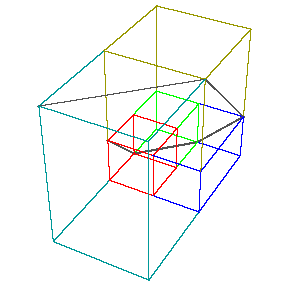top of page
Search

# Padovan sequence, Trinity and Base 3

Updated: Apr 11, 2021

The Padovan sequence is formed out of the trinity 111 and the trinity relates to base 3 and base 3 is formed out of the quantum state of binary and the quantum state can form infinity.

##### The Padovan sequence numbers can be written in terms of powers of the roots of the equation (x^3)-x-1=0. This equation has 3 roots; one real root p (known as the plastic number) and two complex conjugate roots q and r. Given these three roots, the Padovan sequence can be expressed by a formula involving p, q and r:

P(n)=(ap^n)+(bp^n)+(cr^n)

where a, b and c are constants.

Since the magnitudes of the complex roots q and r are both less than 1 (and hence p is a Pisot–Vijayaraghavan number), the powers of these roots approach 0 for large n, and (P(n))-(ap^n) tends to zero.

For all n>0, P(n) is the integer closest to (p^(n-1))/s, where s = p/a = 1.0453567932525329623... is the only real root of s3 − 2s2 + 23s − 23 = 0. The ratio of successive terms in the Padovan sequence approaches p, which has a value of approximately 1.324718. This constant bears the same relationship to the Padovan sequence and the Perrin sequence as the golden ratio does to the Fibonacci sequence.

##### The Padovan sequence forms triangles which form the vibrational dimensions because it forms the Fibonacci spiral out of triangles and the spiral forms the Kathara grid which is encoded in another triangular pattern called Pascal's triangle which we have talked about before and a bigger Kathara grid corresponds to a bigger Pascal's triangle and a bigger one means the membrane is more folded and the starting point of the folding is in the 12th dimension and it unfolds to form the lower dimensions(The membrane encases the Zero-point field/God Source).

1.58 is the dimension of Sierpinski triangle. "Arrowhead construction of the Sierpinski gasket" is basically forming a folding like fractal which forms Sierpinski triangle and this is the fractal shape of the membrane and as we know Sierpinski triangle is formed out of Pascal's triangle which shows a connection between the membrane and Pascals triangle and as we know Pascal's triangle is formed out of the zero-point field.

• e^0.5671432904097838729999686622≈1.75(Ω Constant)

• 1.58/0.5671432904097838729999686622≈2.7

• 2.7=e

Sierpinski triangle base 3 can be formed out of Pascal's triangle mod 3 and the dimension of the Sierpinski triangle is 1.5

e which is 2.7 forms the vibrational dimensions. As shown in a previous post the Sierpinski triangle and Cubistic matrix fractal are related to each other and the Cubistic matrix is the structure of(and encases) the zero-point energy and the Cubistic matrix starts of with 27(2.7) cubes because it is base 3. The Cubistic matrix is formed out of the star tetrahedron which can be formed out of 2 of these membranes merged into one and as we know these membranes fractal, fold more and lots more of them merge into one in higher dimensions.

The Cubistic matrix and the Padovan sequence form the Cuboid spiral which we will talk about next.

##### Cuboid spiral: